# A New Algorithm For Solving Complex Tasks By Partitioning The Reinforcement Learning Task!

3 main points
✔️ Proposes an SIR that can solve complex tasks efficiently by Task Reduction and Self-Imitation
✔️ Effective for sparse reward problems

✔️ Higher performance on manipulation task and maze task than comparative methods

written by  HUazhe XuYilin WuXiaolong WangWi Wu
(Submitted on 26 Jan 2021)## Introduction

Here is a paper that was accepted for publication in ICLR 2021. In recent years, reinforcement learning has become increasingly capable of solving complex tasks, but most methods require carefully designed reward functions. The problem with such reward functions is that they require domain-specific knowledge of the task. Alternatively, there are methods, such as Hierarchical RL (Hierarchical Reinforcement Learning), that learn low-level skills and learn which skills are used by high-level policies. However, learning such low-level skills is not an easy problem. In this article, we introduce a method called Self-Imitation via Reduction (SIR), which can solve sparse reward problems consisting of multiple tasks (compositional structure).

## Technique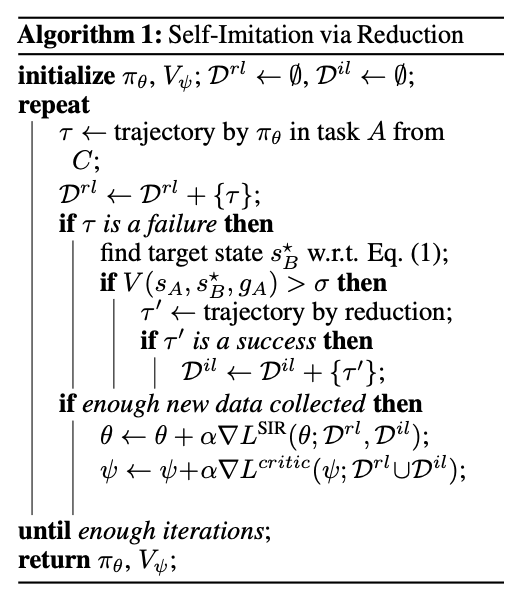Task reduction converts a difficult-to-solve task $A \in \mathcal{T}$ into a task $B \in \mathcal{T}$ that the agent can learn to solve, so that it only needs to solve two simple tasks to solve the original task $A$. In other words, we convert from task $A$ to $B$ and then solve task $B$. To explain this in a little more detail, for the initial state $s_{A}$ and goal $g_{A}$ of the task $A$ that the AGENT cannot solve, we look for a task $B$ that is represented by the initial state $s_{B}$ and goal $g_{b}$ and for which $s_{B}$ can be easily reached from $s_{A}$. Here, the goal $g_{B}$ of task $B$ is the same as the goal $g_{A}$ of task $A$ ($g_{B} = g_{A}$). So how do we measure whether task $B$ is easy or not? This can be done by using the universal value function $V(\cdot)$. In other words, find $s^{\star}_{B}$ such that the following equation is satisfied.

$$s_{B}^{\star}=\arg \max_{s_{B}} V(s_{A}, s_{B}) V(s_{B}, g_{A}):=\arg \max_{s_{b}}V(s_{a}, s_{B}, g_{A})$$

Here, $\oplus$ represents the commposition operator, and there are several choices, minimum, average, product, etc., but in this paper, we use product to denote $V(s_{A}, s_{B}, g_{A})=V(s_{A}, s_{ B})\cdot V(s_{B}, g_{A})$ as follows.

As soon as $s^{\star}_{B}$ is found, the agent runs $\pi(a|s_{A}, s^{\star}_{B})$ from $s^{\star}_{A}$. Then, after reaching the success $s^{'}_{B}$ (i.e. around $s^{\star}_{B}$), $\pi(a|s^{'}_{B}, g_{A})$ is executed to try to reach the final goal. However, the task reduction does not always succeed because either of these two policy executions may fail. There are two possible reasons for the failure, one is the approximation error of the value function, and the second is that task A is simply too difficult for the current policy. Second, we can improve the sample efficiency by making the task reduction only occur when it is likely to be possible to solve the task. In order to do this, we perform task reduction when $V(s_{A}, s^{\star}_{B}, g_{A})$ exceeds a threshold $\sigma$.

### Self-Imitation

If Task reduction is performed on a difficult task $A$ and succeeds in finding $s^{\star}_{B}$, then two trajectories, $s^{'}_{B}$ from $s_{A}$ and $s^{'}_{B}$. from $g_{A}$. Then, by combining these two trajectories, we can obtain a demonstration of the original task. Therefore, by collecting these demonstrations, imitation learning can be applied to these collected self-demonstrations. In this paper, we use the objective function of imitation learning weighted by $A(s, g)$ as follows.

$$L^{il}(\theta; \mathcal{D}^{il})=\mathbb{E}_{(s, a, g) in \mathcal{D}^{il}}[\log \pi (a | s, g)]A(s, g)_{+}$$

Here, $A(s, g)$ represents the clipped advantage, which is computed as $max(0, R-V(s, g))$. This self-imitation allows the agent to solve difficult tasks efficiently.

### SIR: Self-Imitation Via Task Reduction

SIR can be used for both on-policy and off-policy RL. In this paper, we experiment with soft actor-critic (SAC) and proximity policy gradient (PPO). In general, the RL algorithm learns the actor-critic using the collected rollout data $\mathcal{D}^{rl}$. We denote this loss function by $L^{actor}(\theta; \mathcal{D}^{rl})$ and $L^{critic}(psi; \mathcal{D}^{rl})$.

Off-policy RL simply aligns the collected demonstrations with the experience replay data and optimizes the following objective function.

$$L^{SIR}(\theta; \mathcal{D}^{rl}, \mathcal{D}^{il})=L^{actor}(\theta; \mathcal{D}^{rl} \cup \mathcal{D}^{il}) + \beta L^{il}(\theta; \mathcal{D}^{rl} \cup \mathcal{D}^{il})$$

Since on-policy RL ensures that imitation learning is performed on only the collected demonstrations, and since the dataset size of $\mathcal{D}^{il}$ is essentially smaller than the size of $\mathcal{D}^{rl}$, the The following weighted objective function is used for each loss function.

$$L^{SIR}(\theta; \mathcal{D}^{rl}, \mathcal{D}^{il})=\frac{||\mathcal{D}^{rl}||L^{actor}(\theta; \mathcal{D}^{rl}) + ||\mathcal{D}^{il}||L^{il}(\theta; \mathcal{D}^{il})}{||\mathcal{D}^{rl}\cup \mathcal{D}^{il}||}$$

## Experiment

In this experiment, we compared SIR and the baseline method without task reduction in three different MuJoCo simulated environments: the "Push" task, in which the robot pushes objects after clearing obstacles, the "Stack" task, in which the robot stacks objects, and finally the "Maze" task. task in which the robot pushes objects after clearing obstacles, the "Stack" task in which the robot stacks objects, and finally the "Maze" task. All tasks are sparsely rewarded problems, i.e., a $1$ reward is given only if the task is solved, otherwise a $0$ reward is given. As a baseline method, we used SAC for the Push and Stack tasks, and PPO for Maze.

### Push Scenario

As shown in the figure below, there are two objects in the environment, a blue object and a brown elongated object, and the robot has to move the blue object to the location of the red dot. In the figure below, (b) represents the learned value for moving the elongated object, (c) is the learned value for moving the blue object to the goal with respect to different elongated object locations, and (d) is the combined value.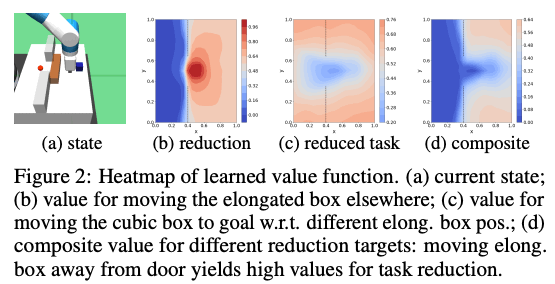The following figures show the results for this task, where the left figure shows the performance when randomly sampled from both difficult and easy tasks, and the right figure shows the performance using only the difficult task. The right figure shows the performance using only difficult tasks. SIL in the lower figure is a combination of SAC and Self-Imitation without task reduction. DS represents the case where SAC is trained with dense reward. From the results below, we can see that SIR is able to solve difficult tasks more efficiently.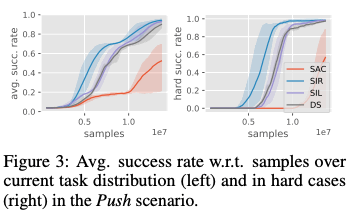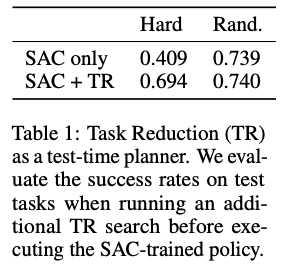### Stack Scenario

In this task, you are given at most three objects of different colors, and you must stack the objects so that the object with the same color as the target reaches the target. In this task, the number of objects, their initial positions, and their colors are randomly specified for each episode. Since this task itself is very difficult, we added a pick-and-place task as a supplementary task, so that at first 70% of the task is solved by pick-and-place and the rest is solved by stack task. The percentage of the pick-and-place task will be reduced as the learning progresses, and eventually it will be reduced to 30% so that the students can learn this task.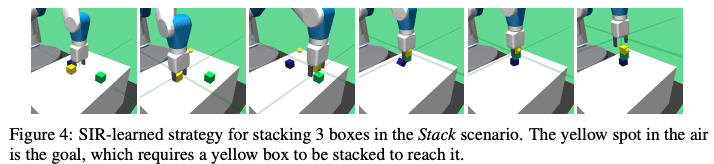As can be seen from the figure below, the proposed method, SIR, successfully solves the task with high accuracy even when the number of objects is three, while the success rates of other methods decrease as the number of objects increases, especially when the number of objects is three.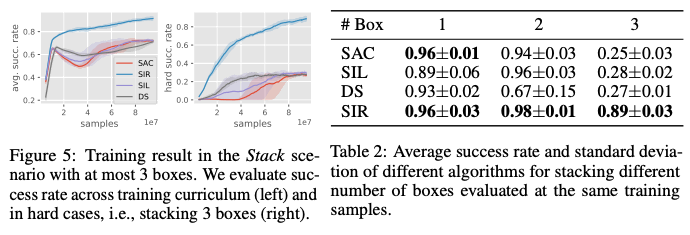### Maze Scenario

In this Maze task, you have to push the green object to the red goal to solve the task. In order to get to the next room, the player has to push the green object to the red goal, and finally, to solve the task, the player has to push the green object to the red goal. A difficult case in this task is when the object has to be moved to another room and at least one door is blocked by a long narrow wall.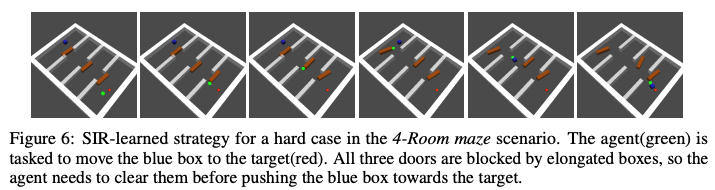In the right graph below, the left graph shows the success rate of the task when all the difficult and easy cases of the task are included, and the right graph shows the result of the difficult case only. As can be seen from the results, the success rate of the proposed method is relatively higher than that of the other methods in the difficult task case, while the success rates of the other methods are lower. In addition, we have trained the proposed method on the U-Wall maze shown in the right figure below. As you can see from the graph on the right side of the figure below, the proposed method, SIR, has the highest success rate in the task, which shows the effectiveness of this method.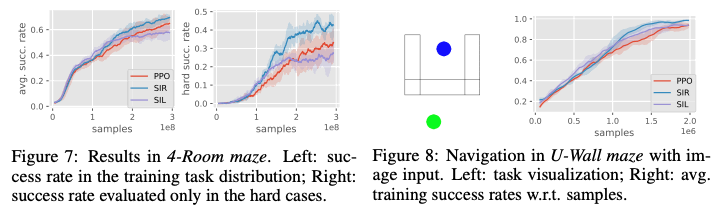In addition, we compared SIR with HIRO, a Hierarchical RL (Hierarchical RL), and DSCtode in the U-shape maze shown in the figure below and in the 3-Room maze with a fixed goal. As a result, as shown in the figure below, SIR has high accuracy and sample efficiency, while the other methods have poor sample efficiency and could not achieve the same success rate.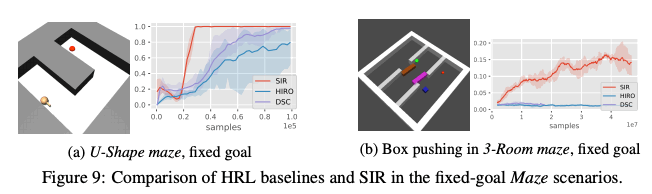### Comparison with Curriculum Learning

The proposed method, SIR, solves an easy task first, and then divides a difficult task into tasks that are already solved by task reduction. Therefore, in the U-maze task with a fixed goal, we compared the proposed method with Goal-GAN, which uses SIR and curriculum learning, and Manual CL, which creates a curriculum manually. From the results shown in the figure below, we can see that the proposed method, SIR, shows a high success rate.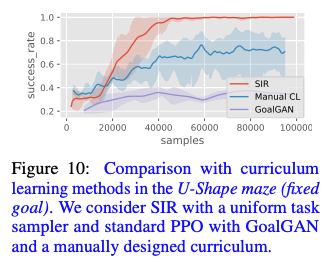## Summary

In this article, we introduced a method called SIR, which splits a difficult task into a problem that has already been solved and a task to be solved by task reduction. RL learns well in relatively simple and clean environments, but not so well in complex environments such as the one in this paper. We believe this is a very important topic because many real-world problems are also complex environments.

If you have any suggestions for improvement of the content of the article,